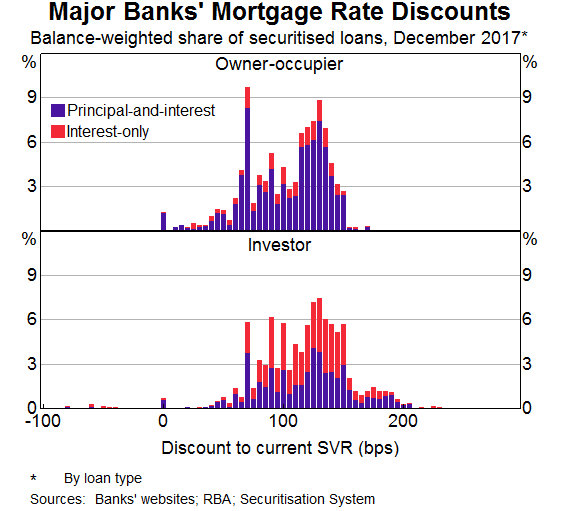Search
•LoanCaddie

# What are interest rate discounts?

An interest rate discounts a margin (quoted in percentage points) that is subtracted from the lenders prevailing standard variable rate to determine the interest payable on your home loan.## What is the range of interest rate discounts?

Based upon a survey undertaken by the RBA, most people are offered an interest rate discount ranging from 0.75%-2.00% for investors and 0.75% -1.50% for owner-occupiers.

Below is a diagram illustrating the distribution of interest rate discounts offered by the major banks.## What are the key factors for determining interest rate discounts?

Based upon analysis undertaken by the RBA, the most significant determinants of interest rate discounts are:

• when the loan was written;

• loan type; and

• loan size.

Of the loans surveyed in December 2017, more recent loans, investors loans and larger loans attracted higher discounts. However, it is now unlikely that investor loan would attract larger discounts given recent bank lending policy changes.

A loan that was written in 2017 typically had a 0.20% larger discount than a similar loan written in 2015.

A loan greater than \$1,000,000 can expect 12bps larger discount than a loan of \$400,000 of the same risk characteristics.

LVR has a minor impact on interest rate discounts. On average, loans with an LVR of 70% only received a 0.10% more discount than loans with an LVR of 90%. However, there are variations between how lenders offer discounts for different LVRs.